Function Repository Resource:

# MagicInteger

Iterate through integer names until an integer equal to its string length is reached

Contributed by: Kevin Reiss and Zach Shelton
 ResourceFunction["MagicInteger"][n] returns a string describing the integer n, then the StringLength of n, etc., until the "magic" integer 4 is reached.

## Details

This game is based off the fact that 4 is the only integer equal to the number of characters in its name. This is often referred to as "4 is magic" or "4 is cosmic."
This function serves as a solution to the Rosetta Code problem Four Is Magic.
Negative inputs are allowed. For example, -9 would be “minus nine.”
The naming convention of integers follows "Cardinal" form from IntegerName.

## Examples

### Basic Examples (2)

4 is magic:

 In:=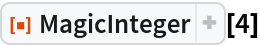Out=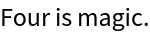"One" has three letters, etc., up until the magic number 4:

 In:=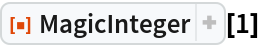Out=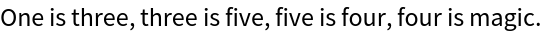### Applications (1)

Find all of the magic numbers below 1000:

 In:=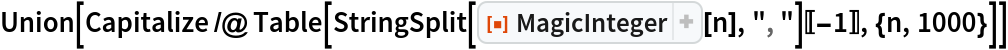Out=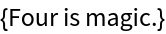### Properties and Relations (1)

Inputs less than 1 can't be magic since every number has more than 0 characters in its name:

 In:=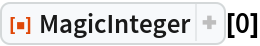Out=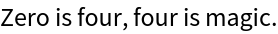In:=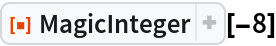Out=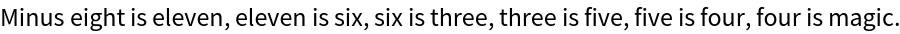### Neat Examples (1)

Find the smallest input under 1000 that has the most iterates by counting the number of commas in the output:

 In:=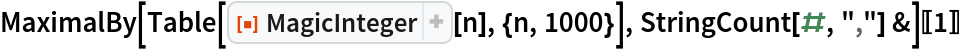Out=Kevin Reiss

## Version History

• 1.0.0 – 28 June 2021